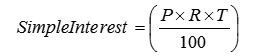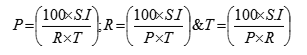# Simple Interest Quantitative Aptitude Interview Questions and Answers Part 1

## SIMPLE INTERESTPRINCIPLE

INTEREST

SIMPLE INTEREST (S.I.)## IMPORTANT FORMULAS FOR SIMPLE INTEREST

1. If P,R,T stand for principle, rate and time respectively and S.I. stands for simple interest interest then,

2. Find simple interest on Rs. 2000 at 5% per annum for 3 years. Also, find the amount?